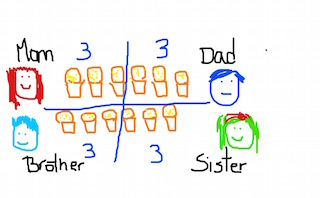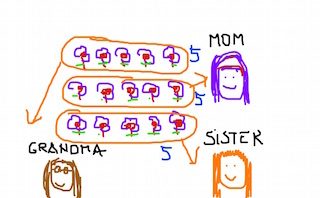# How to Divide By One-Digit Numbers

Instructor: Carla Palomino
Division can make it easy to share things equally with your friends. In this lesson, you will learn the concept of division and an easy way to solve division problems creating your own drawings.

## What Does it Mean to Divide?

Every time you hear the word division, it means something will be split into equal parts or equal groups. The word evenly is often used when we have equal parts.

For example, let's say you have 15 cupcakes, and you want to share them evenly with 3 friends. You will have to divide them among your 3 friends. In order for your friends to get the same amount of cupcakes, they should get 5 each.

## Dividing By One-Digit Numbers

An easy way to help you understand how to divide by one-digit numbers is by drawing. Let's go step by step and solve a few division problems together.

#### Problem 1

A family of four buys a box of ice cream cones that comes with 12 cones. The family needs to divide the ice cream cones evenly between themselves. How many ice cream cones will each of them get?

1. The first thing we will do to solve the problem is draw the situation. Draw the family of 4, and then draw 12 ice cream cones in the middle.

2. Split the ice cream cones among the family. If you give each of them 2, that only makes 8 altogether (2 + 2 + 2 + 2 = 8). But, there are 12 ice cream cones, so you can try to give one more to each of them. Let's try to give them 3 each. That makes 12 altogether (3 + 3 + 3 + 3 = 12) this time, and that's the whole box!#### Problem 2

You bought 15 flowers for your mom, your grandma and your sister. You are planning to split the flowers evenly between the 3 of them. How many flowers will each of them get?

1. Draw the situation (your mom, your grandma and your sister and the flowers in the middle).

2. Split the flowers among them. If you give 4 flowers to each of them, that makes 12 flowers altogether (4 + 4 + 4 = 12). But you bought 15 flowers, so maybe you can give them one more. If you give them 5 flowers each, that makes 15 flowers altogether (5 + 5 + 5 = 15).To unlock this lesson you must be a Study.com Member.

### Register to view this lesson

Are you a student or a teacher?